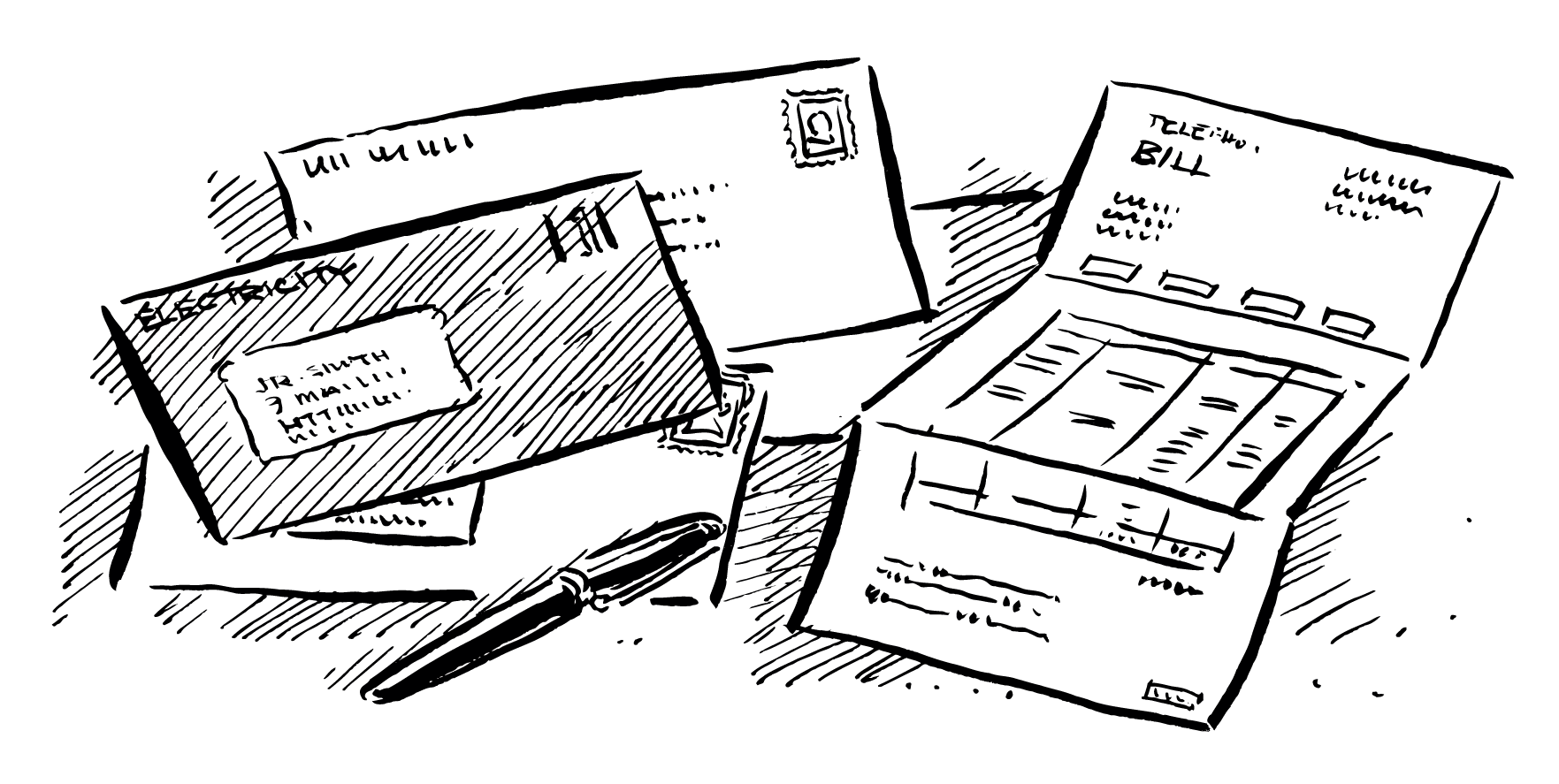CHECK YOUR BILLUse this easy method of adding numbers to check your bank statement or supermarket bill.

The usual way to add numbers works from right to left and so is not useful for mental maths. Numbers are written and spoken from left to right and so it is easier to work from left to right especially when doing it in your head.

• 34 + 52 = 86
This is easy to do from left to right.
We add 3 and 5 to get 8.
And we add 4 and 2 to get 6.

Question Comment !
 Tutorial Title Question Title No Questions 0 Questions Asked 0 Skipped Questions 0 Correctly Answered 0 Wrongly Answered 0 Total Question Attempts 0 Current Question Attempts 0

Skip Question

Reset Test

Halt Test

 No Questions 0 Questions Asked 0 Skipped Questions 0 Correctly Answered 0 Wrongly Answered 0 Total Question Attempts 0

Time Taken

CloseCheck Your Bill (1)

You can use the digit sum method to check these.

Now suppose that there is a carry figure.

• 76 + 86 = 162

The figures on the left add up to 7+8=15.

The figures on the right add up to 6+6=12.

1 5 12 = 1 6 2

Now as 12 is a 2-figure number the 1 will be
carried over to make the 15 into 16, giving 162.

This is easy to do in your head- for any 2-figure number carry the left-hand figure to the left.

• 373 + 474 = 847

First we get 7.
Then we get 14: 714 = 84.
Finally we get 7 which gives 847.Check Your Bill (2)